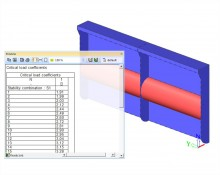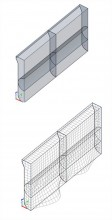# Stability analysis

sens.01

## Highlights

 User input of number of buckling modes to be calculated. The results include buckling factors (ratio between the critical buckling load and the applied load). Deformed shape can be displayed graphically for each calculated buckling mode. The critical buckling mode can be imported in the geometric non-linear calculation as an initial deformation (in combination with geometrical non-linear module)This module calculates the global buckling mode (eigenmode) of a structure under the given loading. In addition, the ratio between the buckling load and the applied load is given. Stability calculations are used to obtain an insight into the buckling mechanisms of a structure, to calculate the buckling length of a member for use in the Steel Code Check, to verify if 2nd order calculations are required. This module helps determine the global critical buckling modes and buckling loads of frame structures as well as surface elements (plates and shells).

Principle of superposition is not valid for a stability calculation. The combinations have to be assembled before starting the calculation. In SCIA Engineer, this is done by defining Stability Combinations. A stability combination is defined as a list of load cases where each load case has a specified coefficient. As specified for non-linear combinations, it is possible to import the linear combinations as stability combinations.

##Following assumptions are used in calculation:

• Physical Linearity
• The elements are taken as ideally straight without any imperfections.
• The loads are guided to the mesh nodes, it is thus mandatory to refine the finite element mesh in order to obtain precise results.
• The critical load coefficient is, per mode, the same for the entire structure.
• Between the mesh nodes, the axial forces and moments are taken as constant.

## Notes

• The first eigenmode is usually the most important and corresponds to the lowest critical load coefficient. A possible collapse of the structure usually happens for this first mode.
• A critical load factor smaller than 1 signifies that the structure is unstable for the given loading.
• Since the calculation searches for eigen values which are close to zero, the calculated l values can be both positive and negative. A negative critical load factor signifies a tensile load. The loading must thus be inversed for buckling to occur (which can for example be the case with wind loads).
• The eigenmodes (buckling shapes) are dimensionless. Only the relative values of the deformations are of importance, the absolute values have no meaning.
• For shell elements the axial force is not considered in one direction only. The shell element can be in compression in one direction and simultaneously in tension in the perpendicular direction. Consequently, the element tends to buckle in one direction but is being ‘stiffened’ in the other direction. This is the reason for significant post-critical bearing capacity of such structures.
• Initial stress is the only local non-linearity taken into account in a linear stability calculation.
• It is important to keep in mind that a stability calculation only examines the theoretical buckling behaviour of the structure. It is thus still required to perform a steel code check to take into account lateral torsional buckling, section checks, combined axial force and bending,…

### Example: Analysis of lateral stability of the building: St Mary of the Angels Primary School - London, United Kingdom; Thomasons

Included in Edition:
Concept
Professional
Expert
Ultimate